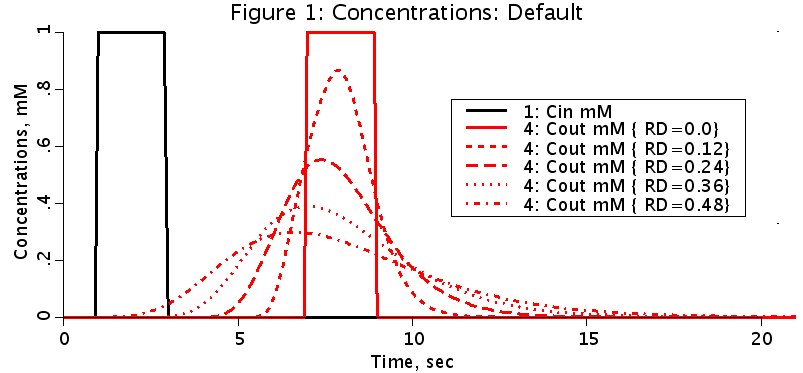# VascularOperator

Model number
0296

Vascular operator consists of a pure delay operator plus two second order operators, all in series to simulate the mean transit time and dispersion in arteries and veins.

## DescriptionFigure shows output concentration when the relative dispersion, RD, is varied from 0.0 to 0.48. This figure matches figure 5A in the reference.

``` This program is for a vascular operator using a pure delay operator and a
4th order operator consisting of two second order operators, all in series.
It is used to simulate the delay and dispersion of a substance passing through
arteries, arterioles, venules, and veins.

Figure shows operator pathways based on RD (relative dispersion input
parameter) value. RD4th is the relative dispersion of a 4th order operator.
RD4th=0.47994 for zeta1=0.95, zeta2=0.8, and wratio = w2/w1 = 1.82.

+--------+
RD=<0   Cin---->| Cdelay |------------------------------------------>Cout
+--------+     A value of zero will be used for RD.

+---------------------------------+
|     4th order operator (op4)    |
+--------+     |   +----------+   +----------+   |
0<RD<RD4th Cin---->| Cdelay |---->|-->| C1 (op2) |-->| C2 (op2) |-->|-->Cout
+--------+     |   +----------|   +----------+   |
+---------------------------------+

+---------------------------------+
|     4th order operator (op4)    |
|   +----------+   +----------+   |
RD=>RD4th Cin---->|-->| C1 (op2) |-->| C2 (op2) |-->|-->Cout
|   +----------|   +----------+   |
+---------------------------------+
A value of RD4th will be used for RD.

The user sets the total delay (totalDelay) and the relative dispersion (RD).
The program calculates a relative dispersion, RD4th, based on the damping
coefficients, zeta1 and zeta2, and the ratio of w2 to w1 (wratio).

2               2      2           2 1/2
(4 zeta2  - 2 + 4 wratio  zeta1  - 2 wratio )
RD4th = ------------------------------------------------.
2 zeta2 + 2 zeta1 wratio

The 4th order operator delay time is
op4Delay = (1-RD/RD4th)*totalDelay.
The pure delay time is
pureDelay = totalDelay-op4Delay.
The relative dispersion of the 4th order operator is RD4th.
The relative dispersion of the pure delay operator is zero.
The relative dispersion of the combined operator is RD.

Cdelay = Cin(t-pureDelay);

The two second order operators solve the following two 2nd order ordinary
differential equations:

/ 2    \
|d     |              /d    \     2
|--- C1| + 2 zeta1 w1 |-- C1| + w1  (C1 - Cdelay) = 0
|  2   |              \dt   /
\dt    /

with initial conditions C1(0)=0 and dC1(0)/dt=0, and

/ 2    \
|d     |              /d    \     2
|--- C2| + 2 zeta2 w2 |-- C2| + w2  (C2 - C1) = 0
|  2   |              \dt   /
\dt    /

with initial conditions C2(0)=0 and dC2(0)/dt=0.

This version allows the user to vary the damping coefficients, zeta2
and zeta2, and wratio, the ratio of w2/w1. zeta1 is protected from
being out of range and RD4th is calculated.```

## Equations

The equations for this model may be viewed by running the JSim model applet and clicking on the Source tab at the bottom left of JSim's Run Time graphical user interface. The equations are written in JSim's Mathematical Modeling Language (MML). See the Introduction to MML and the MML Reference Manual. Additional documentation for MML can be found by using the search option at the Physiome home page.

References
```
R.B. King, A. Deussen, G.M. Raymond, and J.B. Bassinthwaighte. A vascular
transport operator. Am. J. Physiol. 265 (Heart Circ. Physiol. 34): H2196
-H2208, 1993.```
``` Errata

Page H2197: Equation 2 should read
_   _           _
t = t     = t + t   = system V/F
vasc    d   p
_______________________________________________________________________________
Page H2197: The equation given for omega below Figure 3 is incorrect. Correct
1
equation is given in Eq. 11.
_______________________________________________________________________________
Page H2205: The second term in equation T3.1 should be multiplied by 2 to read
2*zeta *omega .
1      1

Page H2205: The second term in equation T3.3 should be multiplied by 2 to read
2*zeta *omega .
2      2
________________________________________________________________________________
Page H2205: The equation for ufreq2 is incorrect. It should read

ufreq2 =(2.0/tmean)*(zeta1*wratio + zeta2)
_______________________________________________________________________________

Page H2206: the equation for ndelay is incorrectly calculated. It should read
ndelay = NINT( (1.0-rd/0.48)*tdelay/dt )```
Key terms
Vascular operator
op2
op4
delay
Paynter
statistics
Acknowledgements

Please cite https://www.imagwiki.nibib.nih.gov/physiome in any publication for which this software is used and send one reprint to the address given below:
The National Simulation Resource, Director J. B. Bassingthwaighte, Department of Bioengineering, University of Washington, Seattle WA 98195-5061.

Model development and archiving support at https://www.imagwiki.nibib.nih.gov/physiome provided by the following grants: NIH U01HL122199 Analyzing the Cardiac Power Grid, 09/15/2015 - 05/31/2020, NIH/NIBIB BE08407 Software Integration, JSim and SBW 6/1/09-5/31/13; NIH/NHLBI T15 HL88516-01 Modeling for Heart, Lung and Blood: From Cell to Organ, 4/1/07-3/31/11; NSF BES-0506477 Adaptive Multi-Scale Model Simulation, 8/15/05-7/31/08; NIH/NHLBI R01 HL073598 Core 3: 3D Imaging and Computer Modeling of the Respiratory Tract, 9/1/04-8/31/09; as well as prior support from NIH/NCRR P41 RR01243 Simulation Resource in Circulatory Mass Transport and Exchange, 12/1/1980-11/30/01 and NIH/NIBIB R01 EB001973 JSim: A Simulation Analysis Platform, 3/1/02-2/28/07.